# 10.5 Graphing quadratic equations  (Page 4/15)

 Page 4 / 15

Find the intercepts of the parabola $y=9{x}^{2}+12x+4.$

$y\text{:}\phantom{\rule{0.2em}{0ex}}\left(0,4\right);x\text{:}\phantom{\rule{0.2em}{0ex}}\left(-\frac{2}{3},0\right)$

## Graph quadratic equations in two variables

Now, we have all the pieces we need in order to graph a quadratic equation in two variables. We just need to put them together. In the next example, we will see how to do this.

## How to graph a quadratic equation in two variables

Graph $y={x}^{2}-6x+8$ .

## Solution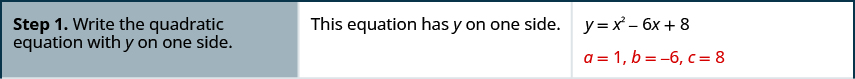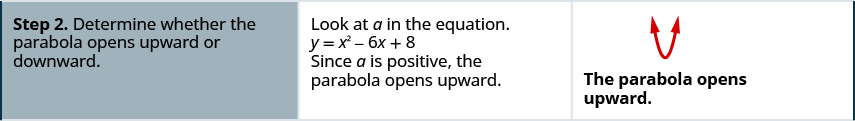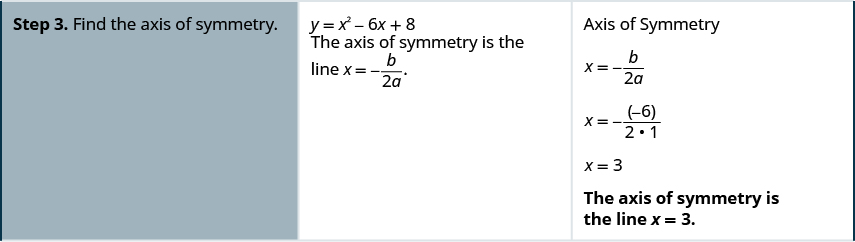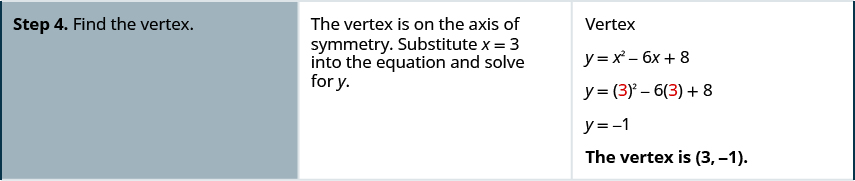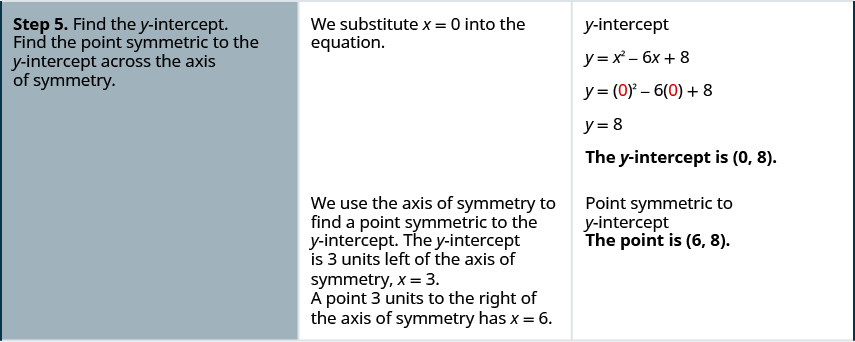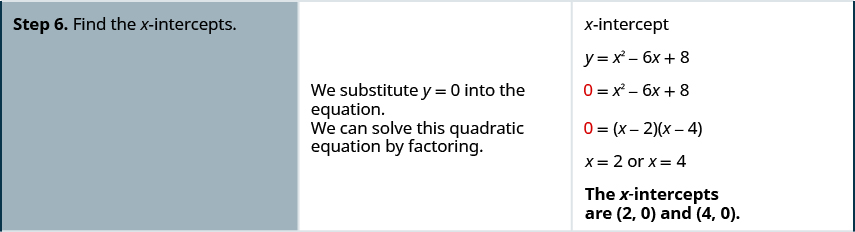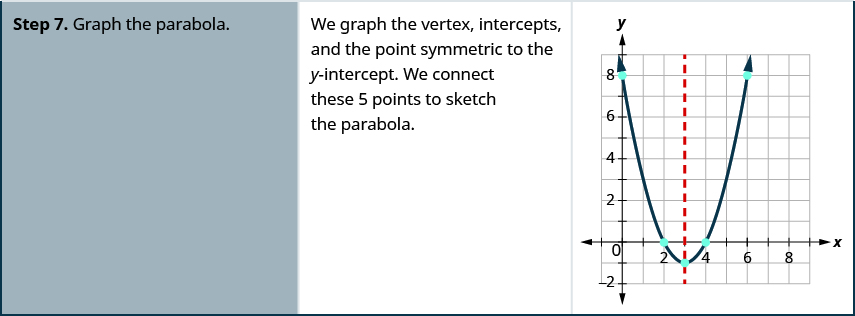Graph the parabola $y={x}^{2}+2x-8.$

$y\text{:}\phantom{\rule{0.2em}{0ex}}\left(0,-8\right)$ ; $x\text{:}\phantom{\rule{0.2em}{0ex}}\left(2,0\right),\left(-4,0\right)$ ;
axis: $x=-1$ ; vertex: $\left(-1,-9\right)$ ;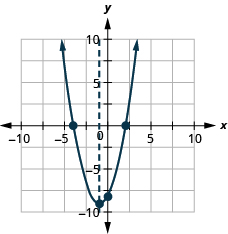Graph the parabola $y={x}^{2}-8x+12.$

$y\text{:}\phantom{\rule{0.2em}{0ex}}\left(0,12\right);\phantom{\rule{0.2em}{0ex}}x\text{:}\phantom{\rule{0.2em}{0ex}}\left(2,0\right),\left(6,0\right);$
axis: $x=4;\phantom{\rule{0.2em}{0ex}}\text{vertex:}\phantom{\rule{0.2em}{0ex}}\left(4,-4\right)$ ;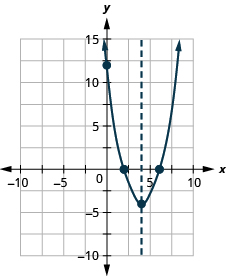## Graph a quadratic equation in two variables.

1. Write the quadratic equation with $y$ on one side.
2. Determine whether the parabola opens upward or downward.
3. Find the axis of symmetry.
4. Find the vertex.
5. Find the y -intercept. Find the point symmetric to the y -intercept across the axis of symmetry.
6. Find the x -intercepts.
7. Graph the parabola.

We were able to find the x -intercepts in the last example by factoring. We find the x -intercepts in the next example by factoring, too.

Graph $y=\text{−}{x}^{2}+6x-9$ .

## Solution

 The equation y has on one side.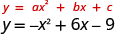Since a is $-1$ , the parabola opens downward. To find the axis of symmetry, find $x=-\frac{b}{2a}$ .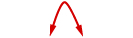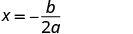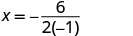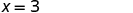The axis of symmetry is $x=3.$ The vertex is on the line $x=3.$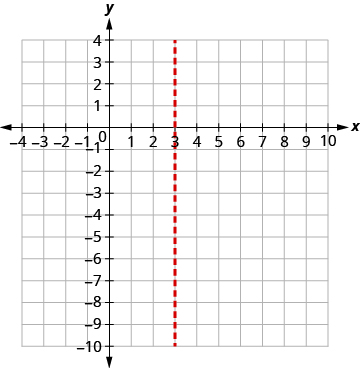Find y when $x=3.$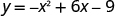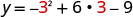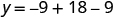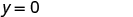The vertex is $\left(3,0\right).$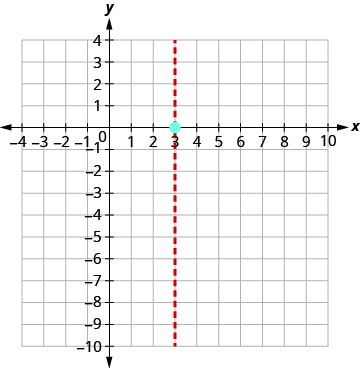The y -intercept occurs when $x=0.$ Substitute $x=0.$ Simplify. The point $\left(0,-9\right)$ is three units to the left of the line of symmetry. The point three units to the right of the line of symmetry is $\left(6,-9\right).$ Point symmetric to the y- intercept is $\left(6,-9\right)$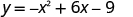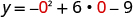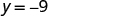The y -intercept is $\left(0,-9\right).$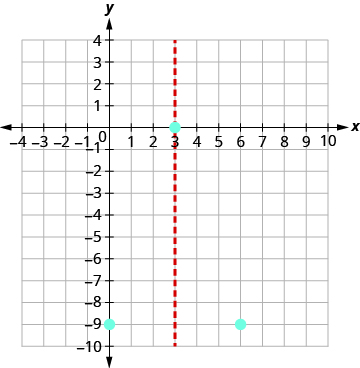The x -intercept occurs when $y=0.$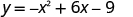Substitute $y=0.$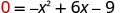Factor the GCF.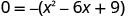Factor the trinomial.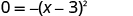Solve for x .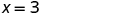Connect the points to graph the parabola.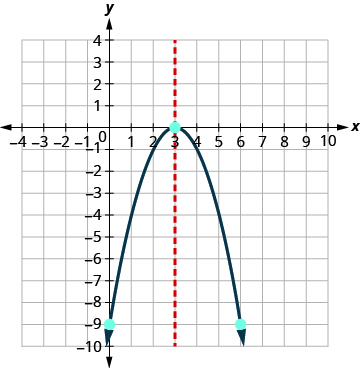Graph the parabola $y=-3{x}^{2}+12x-12.$

$y\text{:}\phantom{\rule{0.2em}{0ex}}\left(0,-12\right);\phantom{\rule{0.2em}{0ex}}x\text{:}\phantom{\rule{0.2em}{0ex}}\left(2,0\right);$
axis: $x=2;\phantom{\rule{0.2em}{0ex}}\text{vertex:}\left(2,0\right)$ ;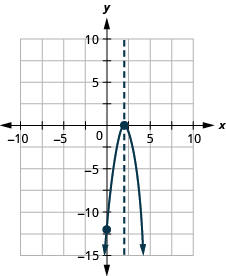Graph the parabola $y=25{x}^{2}+10x+1.$

$y\text{:}\phantom{\rule{0.2em}{0ex}}\left(0,1\right);\phantom{\rule{0.2em}{0ex}}x\text{:}\phantom{\rule{0.2em}{0ex}}\left(-\frac{1}{5},0\right);$
axis: $x=-\frac{1}{5};\phantom{\rule{0.2em}{0ex}}\text{vertex:}\phantom{\rule{0.2em}{0ex}}\left(-\frac{1}{5},0\right)$ ;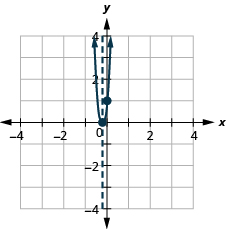For the graph of $y=-{x}^{2}+6x-9$ , the vertex and the x -intercept were the same point. Remember how the discriminant determines the number of solutions of a quadratic equation? The discriminant of the equation $0=\text{−}{x}^{2}+6x-9$ is 0, so there is only one solution. That means there is only one x -intercept, and it is the vertex of the parabola.

How many x -intercepts would you expect to see on the graph of $y={x}^{2}+4x+5$ ?

Graph $y={x}^{2}+4x+5$ .

## Solution

 The equation has y on one side.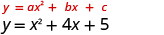Since a is 1, the parabola opens upward.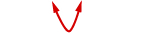To find the axis of symmetry, find $x=-\frac{b}{2a}.$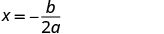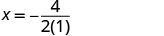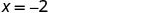The axis of symmetry is $x=-2.$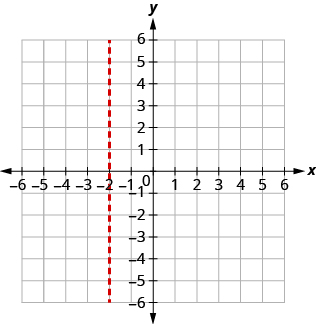The vertex is on the line $x=-2.$ Find y when $x=-2.$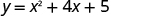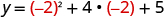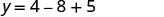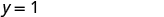The vertex is $\left(-2,1\right).$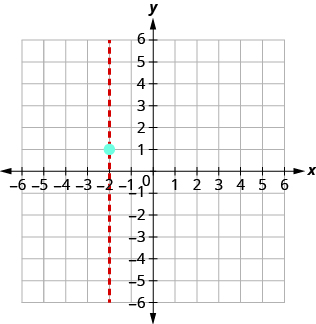The y -intercept occurs when $x=0.$ Substitute $x=0.$ Simplify. The point $\left(0,5\right)$ is two units to the right of the line of symmetry. The point two units to the left of the line of symmetry is $\left(-4,5\right).$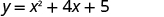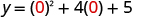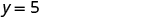The y -intercept is $\left(0,5\right).$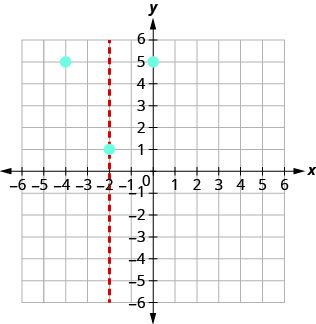Point symmetric to the y- intercept is $\left(-4,5\right)$ . The x - intercept occurs when $y=0.$ Substitute $y=0.$ Test the discriminant.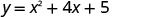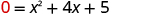${b}^{2}-4ac$ ${4}^{2}-4\cdot 15$ $16-20$ $\phantom{\rule{1em}{0ex}}-4$ Since the value of the discriminant is negative, there is no solution and so no x- intercept. Connect the points to graph the parabola. You may want to choose two more points for greater accuracy.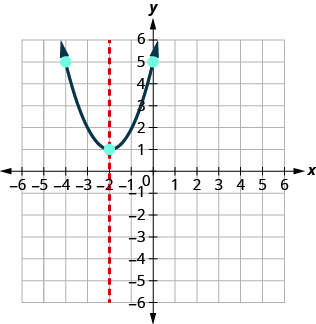Graph the parabola $y=2{x}^{2}-6x+5.$

$y\text{:}\phantom{\rule{0.2em}{0ex}}\left(0,5\right);\phantom{\rule{0.2em}{0ex}}x\text{:}\phantom{\rule{0.2em}{0ex}}\text{none};$
axis: $x=\frac{3}{2};\phantom{\rule{0.2em}{0ex}}\text{vertex:}\phantom{\rule{0.2em}{0ex}}\left(\frac{3}{2},\frac{1}{2}\right)$ ;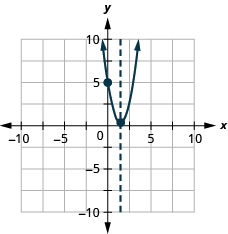Graph the parabola $y=-2{x}^{2}-1.$

$y\text{:}\phantom{\rule{0.2em}{0ex}}\left(0,-1\right);x\text{:}\phantom{\rule{0.2em}{0ex}}\text{none};$
axis: $x=0;\phantom{\rule{0.2em}{0ex}}\text{vertex:}\phantom{\rule{0.2em}{0ex}}\left(0,-1\right)$ ;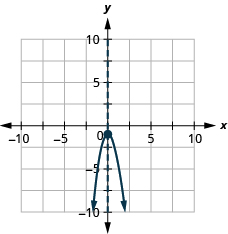Macario is making 12 pounds of nut mixture with macadamia nuts and almonds. macadamia nuts cost $9 per pound and almonds cost$5.25 per pound. how many pounds of macadamia nuts and how many pounds of almonds should macario use for the mixture to cost $6.50 per pound to make? Cherry Reply Nga and Lauren bought a chest at a flea market for$50. They re-finished it and then added a 350 % mark - up
the sum of two Numbers is 19 and their difference is 15
2, 17
Jose
interesting
saw
Felecia left her home to visit her daughter, driving 45mph. Her husband waited for the dog sitter to arrive and left home 20 minutes, or 13 hour later. He drove 55mph to catch up to Felecia. How long before he reaches her?
integer greater than 2 and less than 12
2 < x < 12
Felix
I'm guessing you are doing inequalities...
Felix
Actually, translating words into algebraic expressions / equations...
Felix
hi
Darianna
hello
Mister
Eric here
Eric
He charges $125 per job. His monthly expenses are$1,600. How many jobs must he work in order to make a profit of at least $2,400? Alicia Reply at least 20 Ayla what are the steps? Alicia 6.4 jobs Grahame 32 Grahame 1600+2400= total amount with expenses. 4000/125= number of jobs needed to make that min profit of 2400. answer is 32 Orlando He must work 32 jobs to make a profit POP what is algebra Azhar Reply repeated addition and subtraction of the order of operations. i love algebra I'm obsessed. Shemiah hi Krekar Eric here. I'm a parent. 53 years old. I have never taken algebra. I want to learn. Eric One-fourth of the candies in a bag of M&M’s are red. If there are 23 red candies, how many candies are in the bag? Leanna Reply they are 92 candies in the bag POP rectangular field solutions Navin Reply What is this? Donna t muqtaar the proudact of 3x^3-5×^2+3 and 2x^2+5x-4 in z7[x]/ is anas Reply ? Choli a rock is thrown directly upward with an initial velocity of 96feet per second from a cliff 190 feet above a beach. The hight of tha rock above the beach after t second is given by the equation h=_16t^2+96t+190 Usman Stella bought a dinette set on sale for$725. The original price was $1,299. To the nearest tenth of a percent, what was the rate of discount? Manhwa Reply 44.19% Scott 40.22% Terence 44.2% Orlando I don't know Donna if you want the discounted price subtract$725 from $1299. then divide the answer by$1299. you get 0.4419... but as percent you get 44.19... but to the nearest tenth... round .19 to .2 and you get 44.2%
Orlando
you could also just divide $725/$1299 and then subtract it from 1. then you get the same answer.
Orlando
p mulripied-5 and add 30 to it
Tausif
Tausif
how
muqtaar
Can you explain further
p mulripied-5 and add to 30
Tausif
-5p+30?
Corey
p=-5+30
Jacob
How do you find divisible numbers without a calculator?
TAKE OFF THE LAST DIGIT AND MULTIPLY IT 9. SUBTRACT IT THE DIGITS YOU HAVE LEFT. IF THE ANSWER DIVIDES BY 13(OR IS ZERO), THEN YOUR ORIGINAL NUMBER WILL ALSO DIVIDE BY 13!IS DIVISIBLE BY 13
BAINAMA
When she graduates college, Linda will owe $43,000 in student loans. The interest rate on the federal loans is 4.5% and the rate on the private bank loans is 2%. The total interest she owes for one year was$1,585. What is the amount of each loan?By OpenStaxBy OpenStaxBy Keyaira BraxtonBy OpenStaxBy Stephen VoronBy David CoreyByBy OpenStaxByBy Ryan Lowe